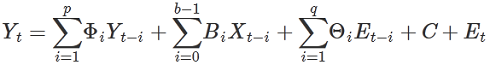# 1.0 - 8.00 - VARMAX - Teradata Vantage

## Teradata® Vantage Machine Learning Engine Analytic Function Reference

Product
Release Number
1.0
8.00
Release Date
May 2019
Content Type
Programming Reference
Publication ID
B700-4003-098K
Language
English (United States)

VARMAX (Vector Autoregressive Moving Average model with eXogenous variables) extends the ARMA/ARIMA model in two ways:

• To work with time series with multiple response variables (vector time series).
• To work with exogenous variables, or variables that are independent of the other variables in the system.

The model includes both the dynamic relationship between the multiple response variables and the relationship between the dependent and independent variables.

## Nonseasonal VARMAX Model

This formula represents a nonseasonal VARMAX model:Variable Description
Y t Time series made stationary. First term is autoregressive component, second term is exogenous component, third term is moving average component, the fourth term (C) is vector of constants, and fifth term (E t ) is vector of residual errors.
Y t Vector of n response variables.
X t Vector of m exogenous variables.
p Number of previous periods of endogenous variables included in model.
q Number of previous periods included in moving average.
b Number of previous periods of exogenous variables included.
Φ i n * n matrix of autoregressive parameters.
B i n * m matrix of exogenous variable parameters.
Θ i n * n matrix of moving average parameters.
E t Difference between actual and predicted value of Y t , (Y t - Ŷ t ).

## Seasonal VARMAX Model

This formula represents a seasonal VARMAX model:

(1 - Φ1Back - … - Φ p Back p )(1 - Φ1Back m - … - Φ sp Back m*sp )(1 - Back) d (1 - Back m ) sd (Y t ) =

C + (1 + Θ1Back + … + Θ q Back q ) (1 + Θ1Back m + … + Θ sq Back m*sq ) E t +

(B 1 + B 2Back + … + B b-1Back b ) (1 - Back) d (1 - Back m ) sd X t

Variables are as in the nonseasonal VARMAX Model and the following table.

Variable Description
Back Back-shift operator, that is:

Back(y t ) = y t-1

m Number of periods in each season.
d Number of differencing steps performed to stationarize time series.
sp Seasonal parameter corresponding to p.
sq Seasonal parameter corresponding to q.
sd Seasonal parameter corresponding to d.

## Difference between VARMAX and R Function VARMA

The results of the VARMAX function and the VARMA function in the R package MTS might not match exactly, because VARMA applies a boundary to the numerical optimization and VARMAX does not. Also, the default optimization method (the Method argument) is different for VARMA and VARMAX.